# QDagger

## Overview

QDagger is an extension of the DQN algorithm that uses previously computed results, like teacher policy and teacher replay buffer, to help train student policy. This method eliminates the need for learning from scratch, improving sample efficiency and reducing computational effort in training new policy.

Original paper:

Reference resources:

## Implemented Variants

Variants Implemented Description
qdagger_dqn_atari_impalacnn.py, docs For playing Atari games. It uses Impala-CNN from RainbowDQN and common atari-based pre-processing techniques.
qdagger_dqn_atari_jax_impalacnn.py, docs For playing Atari games. It uses Impala-CNN from RainbowDQN and common atari-based pre-processing techniques.

Below are our single-file implementations of QDagger:

## qdagger_dqn_atari_impalacnn.py

The qdagger_dqn_atari_impalacnn.py has the following features:

• For playing Atari games. It uses Impala-CNN from RainbowDQN and common atari-based pre-processing techniques.
• Its teacher policy uses CleanRL's dqn_atari policy from the huggingface/cleanrl repository.
• Works with the Atari's pixel Box observation space of shape (210, 160, 3)
• Works with the Discrete action space

### Usage

poetry install -E atari
python cleanrl/qdagger_dqn_atari_impalacnn.py --env-id BreakoutNoFrameskip-v4
python cleanrl/qdagger_dqn_atari_impalacnn.py --env-id PongNoFrameskip-v4

poetry install -E atari
poetry run python cleanrl/qdagger_dqn_atari_impalacnn.py --env-id BreakoutNoFrameskip-v4
poetry run python cleanrl/qdagger_dqn_atari_impalacnn.py --env-id PongNoFrameskip-v4

pip install -r requirements/requirements-atari.txt
python cleanrl/qdagger_dqn_atari_impalacnn.py --env-id BreakoutNoFrameskip-v4
python cleanrl/qdagger_dqn_atari_impalacnn.py --env-id PongNoFrameskip-v4


### Explanation of the logged metrics

Running python cleanrl/qdagger_dqn_atari_impalacnn.py will automatically record various metrics such as value or distillation losses in Tensorboard. Below is the documentation for these metrics:

• charts/episodic_return: episodic return of the game
• charts/SPS: number of steps per second
• losses/td_loss: the mean squared error (MSE) between the Q values at timestep $$t$$ and the Bellman update target estimated using the reward $$r_t$$ and the Q values at timestep $$t+1$$, thus minimizing the one-step temporal difference. Formally, it can be expressed by the equation below. $$J(\theta^{Q}) = \mathbb{E}_{(s,a,r,s') \sim \mathcal{D}} \big[ (Q(s, a) - y)^2 \big],$$ with the Bellman update target is $$y = r + \gamma \, Q^{'}(s', a')$$ and the replay buffer is $$\mathcal{D}$$.
• losses/q_values: implemented as qf1(data.observations, data.actions).view(-1), it is the average Q values of the sampled data in the replay buffer; useful when gauging if under or over estimation happens.
• losses/distill_loss: the distillation loss, which is the KL divergence between the teacher policy $$\pi_T$$ and the student policy $$\pi$$. Formally, it can be expressed by the equation below. $$L_{\text{distill}} = \lambda_t \mathbb{E}_{(s,a,r,s') \sim \mathcal{D}} \left[ \sum_a \pi_T(a|s)\log\pi(a|s)\right]$$
• Charts/distill_coeff: the coefficient $$\lambda_t$$ for the distillation loss, which is a function of the ratio between the teacher policy $$\pi_T$$ and the student policy $$\pi$$. Formally, it can be expressed by the equation below. $$\lambda_t = 1_{t<t_0}\max(1 - G^\pi/G^{\pi_T}, 0)$$
• losses/loss: the total loss, which is the sum of the TD loss and the distillation loss. $$L_{\text{qdagger}} = J(\theta^{Q}) + L_{\text{distill}}$$
• charts/teacher/avg_episodic_return: average episodic return of teacher policy evaluation
• charts/offline/avg_episodic_return: average episodic return of policy evaluation in offline training phase

### Implementation details

qdagger_dqn_atari_impalacnn.py is based on (Agarwal et al., 2022)1 but presents a few implementation differences:

• (Agarwal et al., 2022)1 uses the teacher replay buffer data saved during the training of the teacher policy, but our teacher policy, which uses CleanRL's dqn_atari from the huggingface/cleanrl, does not contain replay buffer data, so we populate the teacher replay buffer with the teacher policy before training. For more details, see section A.5 "Additional ablations for QDagger" in the original paper.
• (Agarwal et al., 2022)1 uses DQN (Adam) @ 400M frames, but we use CleanRL's dqn_atari, which is DQN (Adam) @ 10M steps(40M frames).
• We have used an old set of Atari preprocessing techniques that doesn't use sticky action, but the original paper does.

### Experiment results

Below are the average episodic returns for qdagger_dqn_atari_impalacnn.py.

Environment qdagger_dqn_atari_impalacnn.py 10M steps(40M frames) (Agarwal et al., 2022)1 10M frames
BreakoutNoFrameskip-v4 295.55 ± 12.30 275.15 ± 20.65
PongNoFrameskip-v4 19.72 ± 0.20 -
BeamRiderNoFrameskip-v4 9284.99 ± 242.28 6514.25 ± 411.10

Learning curves: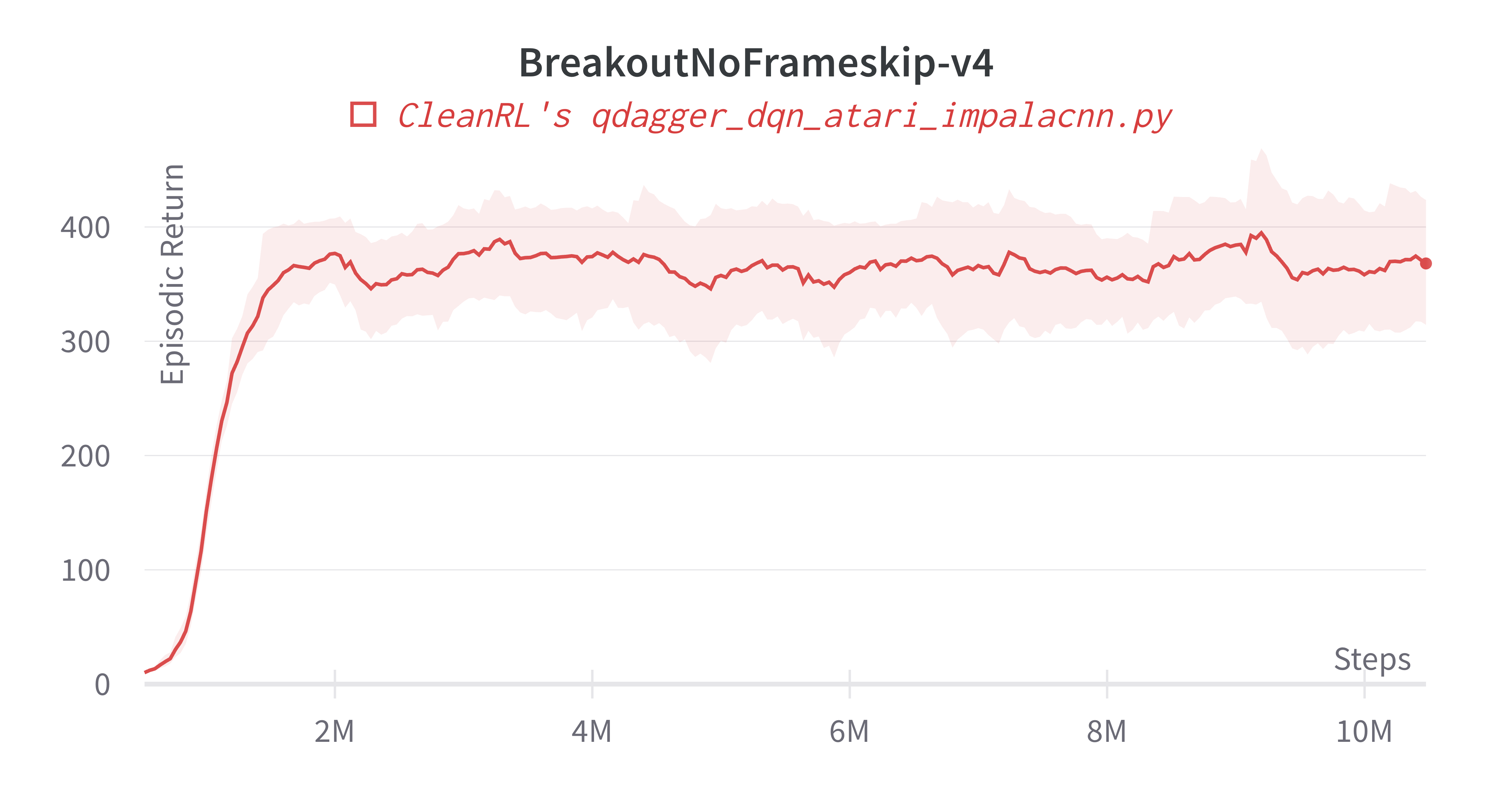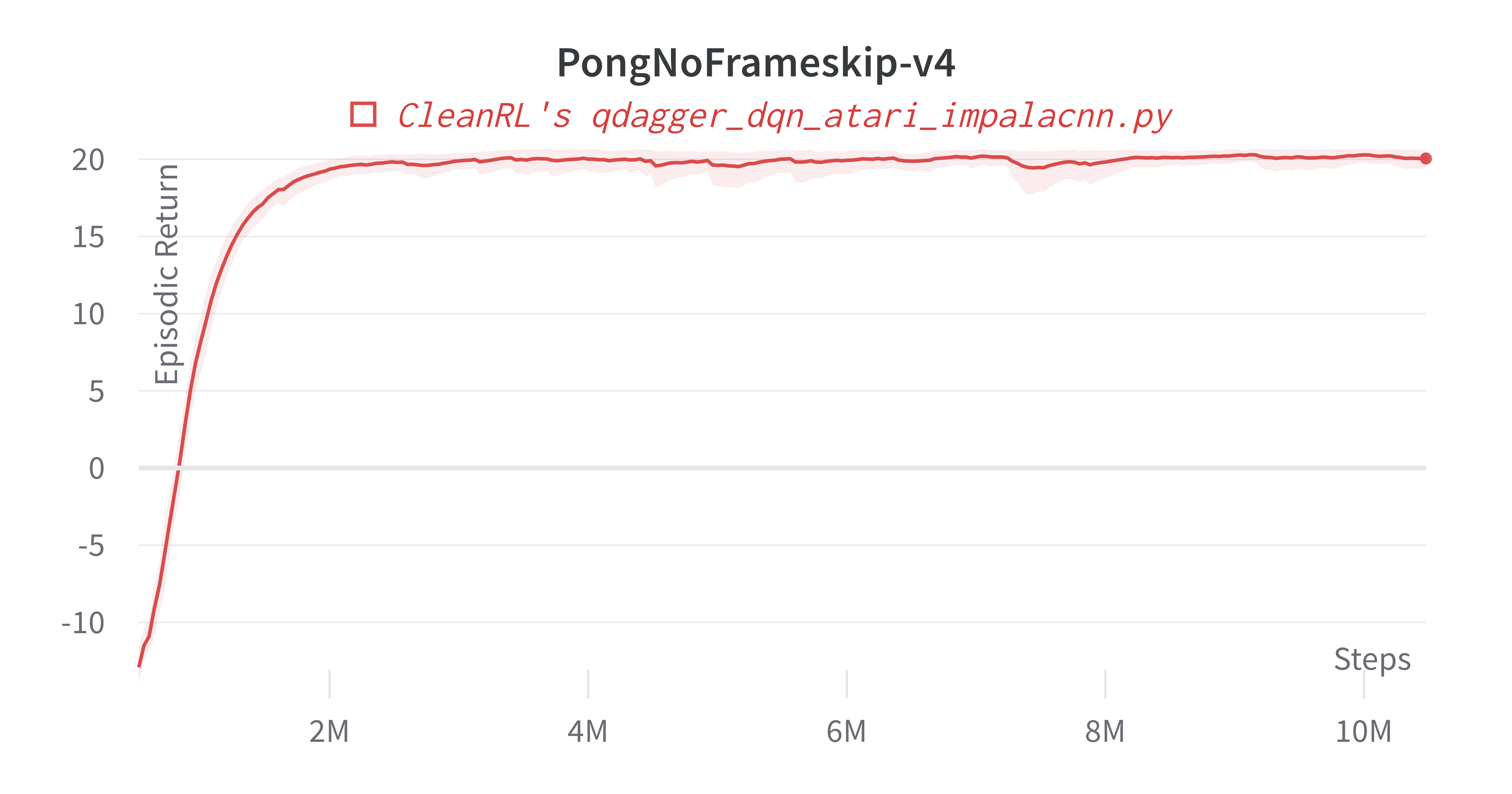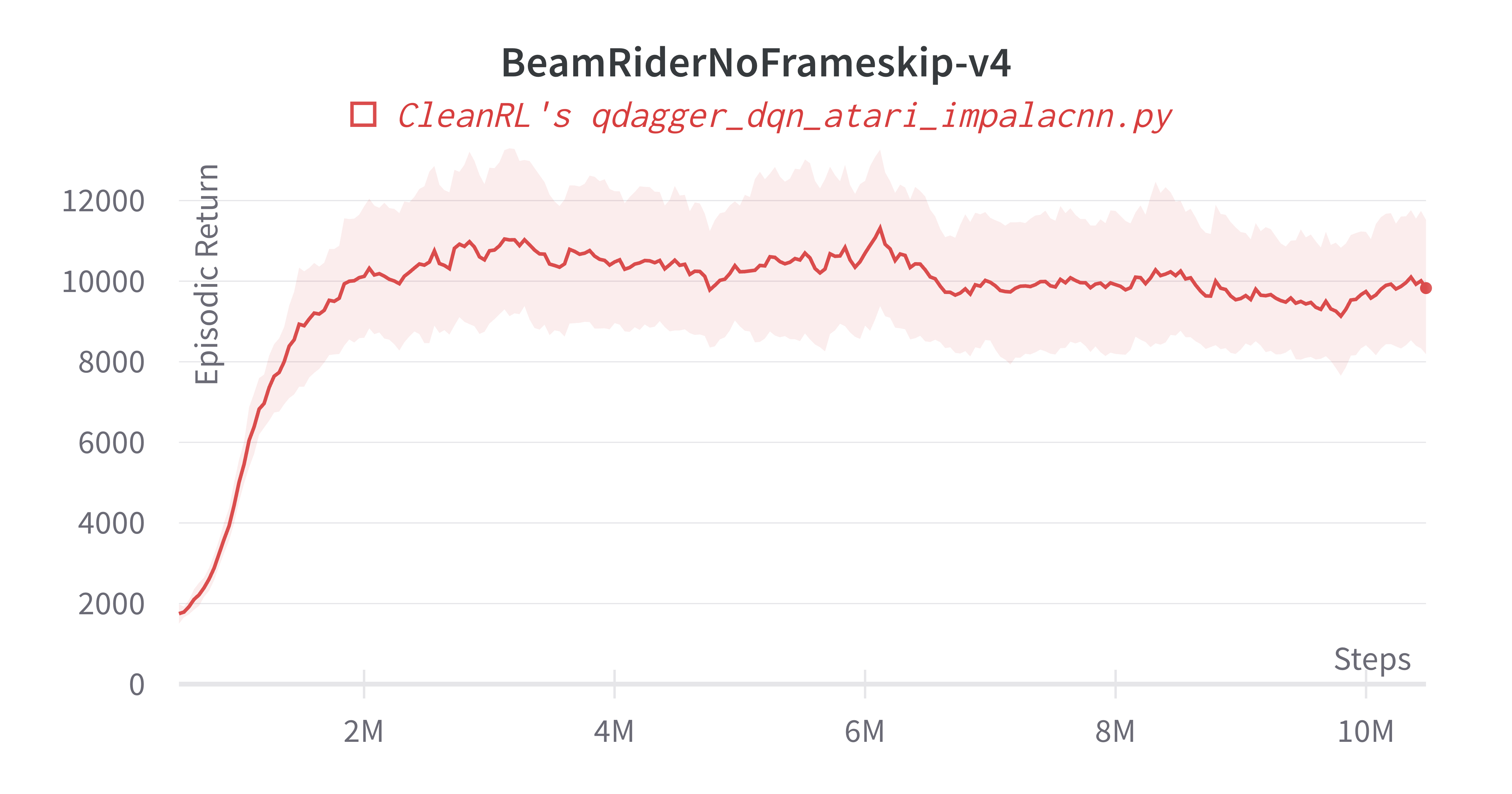Learning curve comparison with dqn_atari: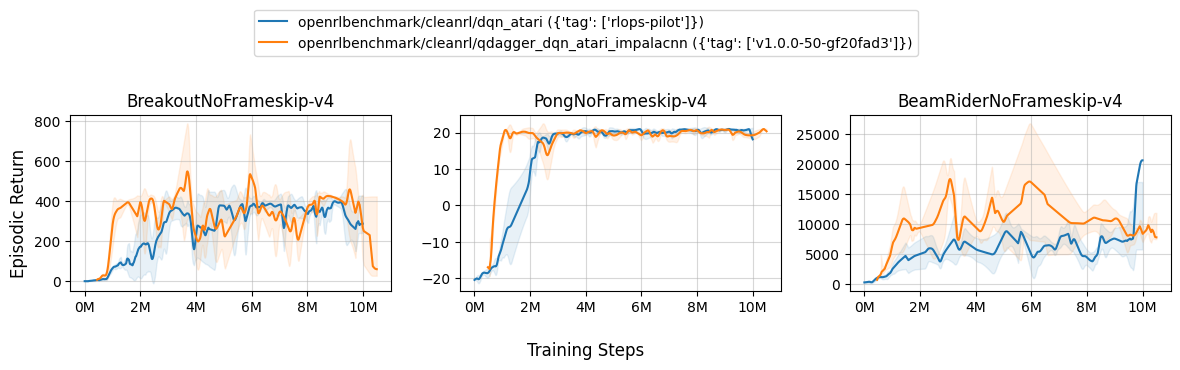Tracked experiments and game play videos:

## qdagger_dqn_atari_jax_impalacnn.py

The qdagger_dqn_atari_jax_impalacnn.py has the following features:

• Uses Jax, Flax, and Optax instead of torch. qdagger_dqn_atari_jax_impalacnn.py is roughly 25%-50% faster than qdagger_dqn_atari_impalacnn.py
• For playing Atari games. It uses Impala-CNN from RainbowDQN and common atari-based pre-processing techniques.
• Its teacher policy uses CleanRL's dqn_atari_jax policy from the huggingface/cleanrl repository.
• Works with the Atari's pixel Box observation space of shape (210, 160, 3)
• Works with the Discrete action space

### Usage

poetry install -E "atari jax"
poetry run python cleanrl/qdagger_dqn_atari_jax_impalacnn.py --env-id BreakoutNoFrameskip-v4
poetry run python cleanrl/qdagger_dqn_atari_jax_impalacnn.py --env-id PongNoFrameskip-v4

pip install -r requirements/requirements-atari.txt
pip install -r requirements/requirements-jax.txt
python cleanrl/qdagger_dqn_atari_jax_impalacnn.py --env-id BreakoutNoFrameskip-v4
python cleanrl/qdagger_dqn_atari_jax_impalacnn.py --env-id PongNoFrameskip-v4

Warning

Note that JAX does not work in Windows . The official docs recommends using Windows Subsystem for Linux (WSL) to install JAX.

### Explanation of the logged metrics

See related docs for qdagger_dqn_atari_impalacnn.py.

### Implementation details

See related docs for qdagger_dqn_atari_impalacnn.py.

### Experiment results

Below are the average episodic returns for qdagger_dqn_atari_jax_impalacnn.py.

Environment qdagger_dqn_atari_jax_impalacnn.py 10M steps(40M frames) (Agarwal et al., 2022)1 10M frames
BreakoutNoFrameskip-v4 335.08 ± 19.12 275.15 ± 20.65
PongNoFrameskip-v4 18.75 ± 0.19 -
BeamRiderNoFrameskip-v4 8024.75 ± 579.02 6514.25 ± 411.10

Learning curves: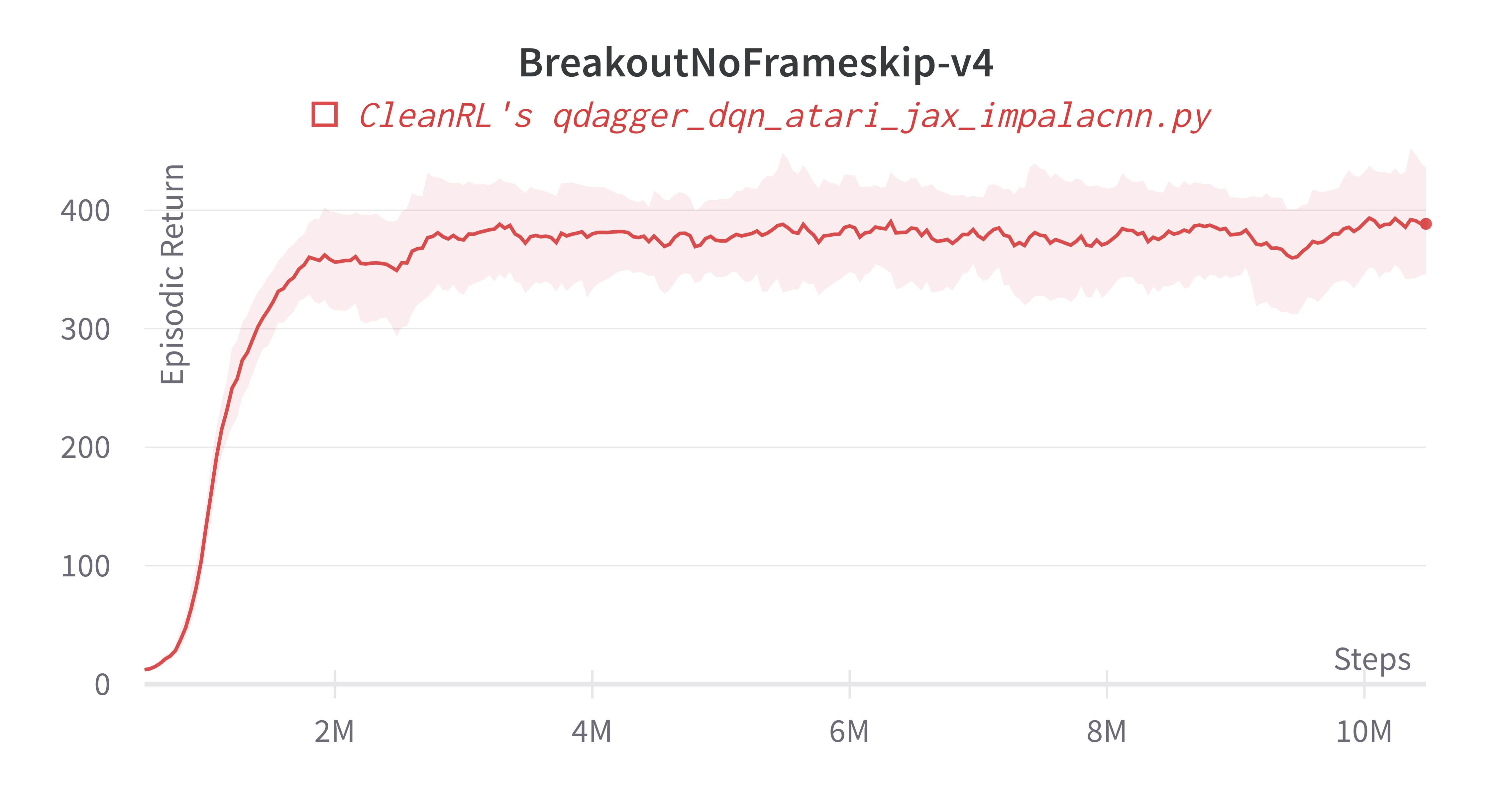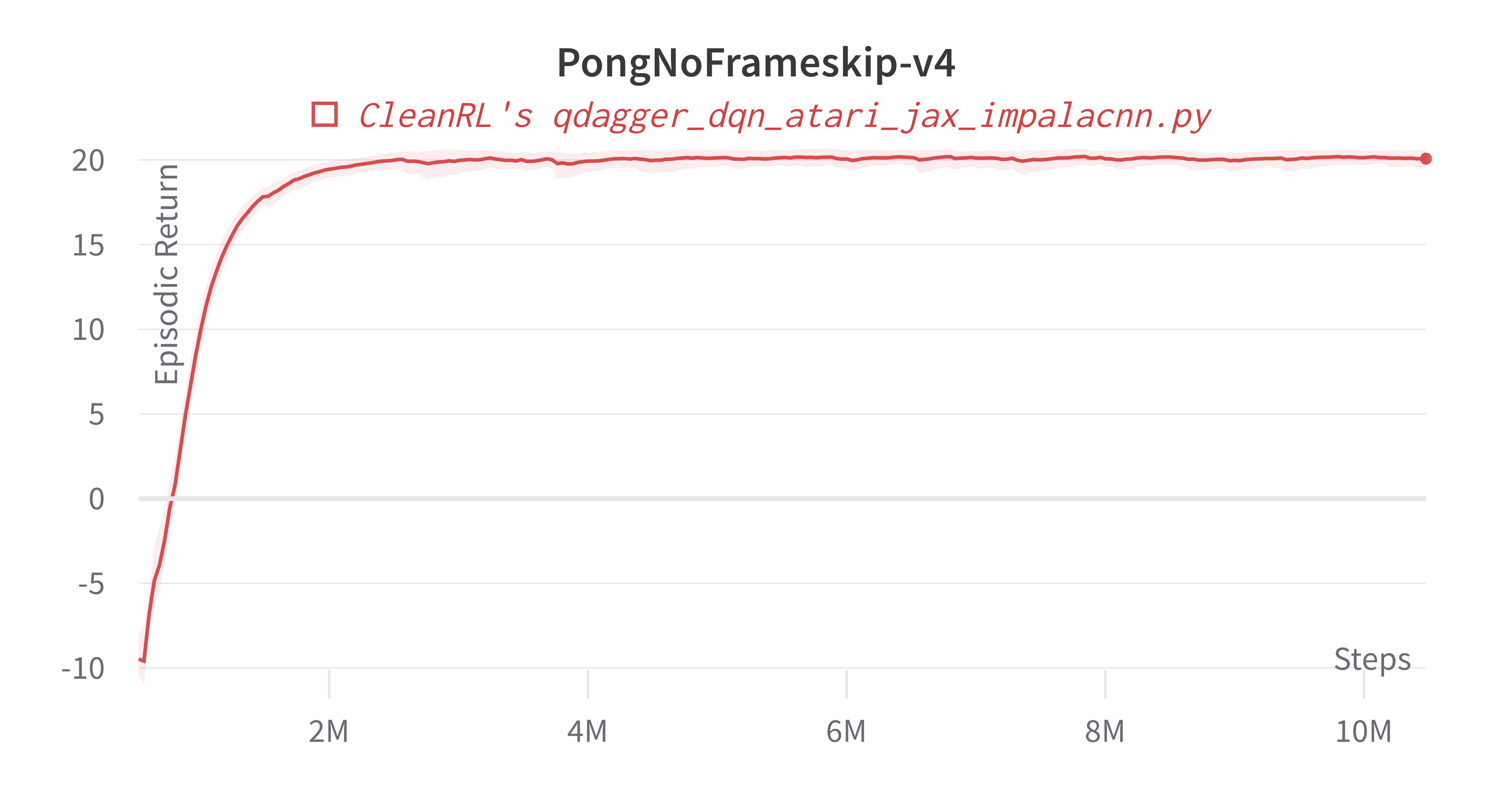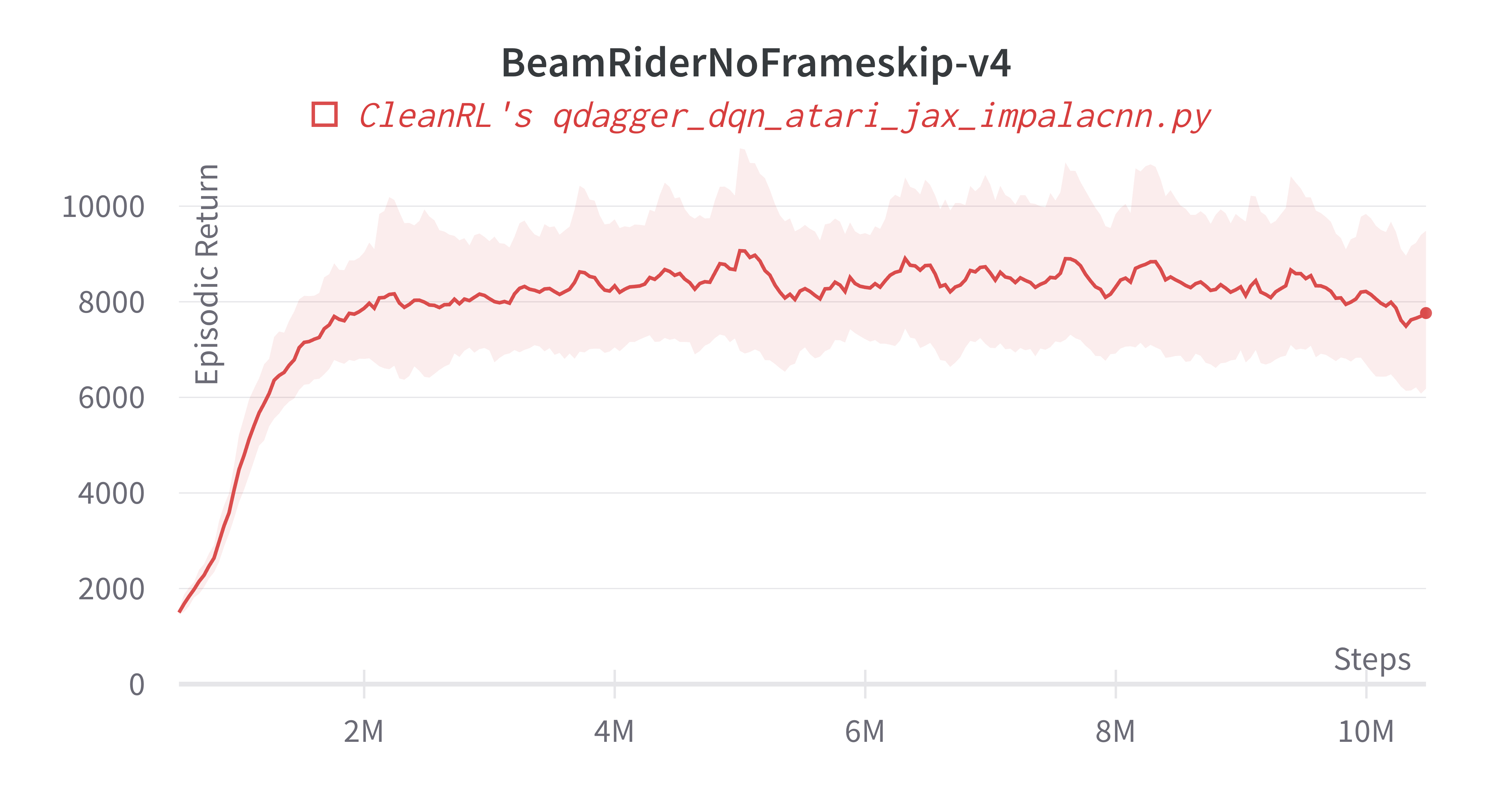Learning curve comparison with dqn_atari_jax: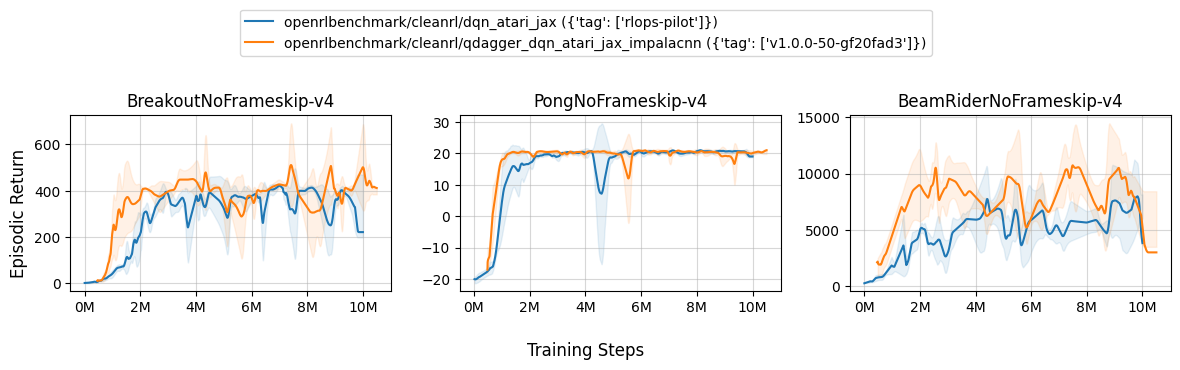1. Agarwal, Rishabh, Max Schwarzer, Pablo Samuel Castro, Aaron Courville, and Marc G. Bellemare. “Reincarnating Reinforcement Learning: Reusing Prior Computation to Accelerate Progress.” arXiv, October 4, 2022. http://arxiv.org/abs/2206.01626.